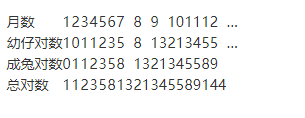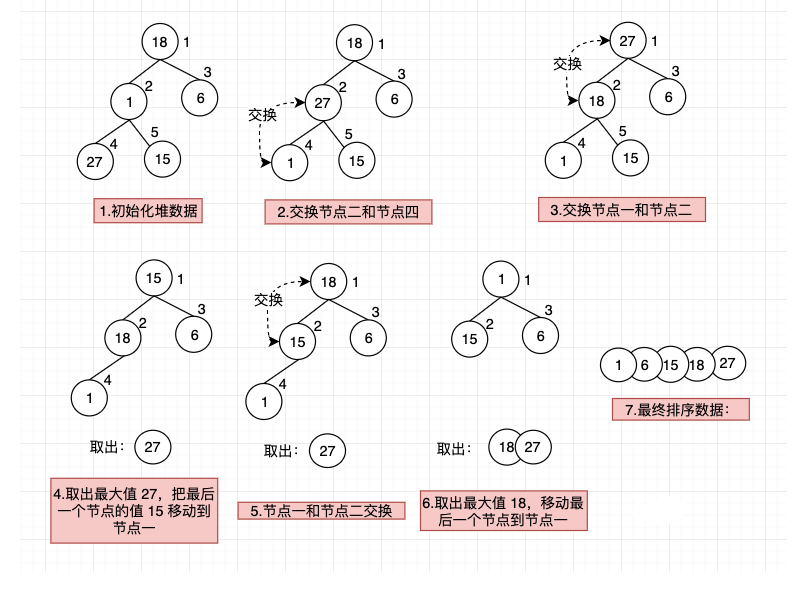# Java笔试面试-算法常用面试题

1.说一下什么是二分法？使用二分法时需要注意什么？如何用代码实现？

二分法查找（Binary Search）也称折半查找，是指当每次查询时，将数据分为前后两部分，再用中值和待搜索的值进行比较，如果搜索的值大于中值，则使用同样的方式（二分法）向后搜索，反之则向前搜索，直到搜索结束为止。
二分法使用的时候需要注意：二分法只适用于有序的数据，也就是说，数据必须是从小到大，或是从大到小排序的。

public class Lesson7_4 {
public static void main(String[] args) {
// 二分法查找
int[] binaryNums = {1, 6, 15, 18, 27, 50};
int findValue = 27;
int binaryResult = binarySearch(binaryNums, 0, binaryNums.length - 1, findValue);
System.out.println("元素第一次出现的位置（从0开始）：" + binaryResult);
}
/**
* 二分查找，返回该值第一次出现的位置（下标从 0 开始）
* @param nums      查询数组
* @param start     开始下标
* @param end       结束下标
* @param findValue 要查找的值
* @return int
*/
private static int binarySearch(int[] nums, int start, int end, int findValue) {
if (start <= end) {
// 中间位置
int middle = (start + end) / 2;
// 中间的值
int middleValue = nums[middle];
if (findValue == middleValue) {
// 等于中值直接返回
return middle;
} else if (findValue < middleValue) {
// 小于中值，在中值之前的数据中查找
return binarySearch(nums, start, middle - 1, findValue);
} else {
// 大于中值，在中值之后的数据中查找
return binarySearch(nums, middle + 1, end, findValue);
}
}
return -1;
}
}


元素第一次出现的位置（从0开始）：4


2.什么是斐波那契数列？用代码如何实现？

斐波那契数列（Fibonacci Sequence），又称黄金分割数列、因数学家列昂纳多·斐波那契（Leonardoda Fibonacci）以兔子繁殖为例子而引入，故又称为“兔子数列”，指的是这样一个数列：0, 1, 1, 2, 3, 5, 8, 13, 21, 34, 55, 89, 144, 233，377，610，987，1597，2584，4181，6765，10946，17711…… 在数学上，斐波那契数列以如下被以递推的方法定义：
F(1)=1，F(2)=1, F(n)=F(n-1)+F(n-2)（n>=3，n∈N*）在现代物理、准晶体结构、化学等领域，斐波纳契数列都有直接的应用。

斐波那契数列之所以又称黄金分割数列，是因为随着数列项数的增加，前一项与后一项之比越来越逼近黄金分割的数值 0.6180339887……

斐波那契数列指的是这样一个数列：0, 1, 1, 2, 3, 5, 8, 13, 21, 34, 55, 89, 144, 233，377，610，987，1597，2584，4181，6765，10946，17711……

斐波那契数列的特征：第三项开始（含第三项）它的值等于前两项之和。

斐波那契数列代码实现示例，如下所示：

public class Lesson7_4 {
public static void main(String[] args) {
// 斐波那契数列
int fibonacciIndex = 7;
int fibonacciResult = fibonacci(fibonacciIndex);
System.out.println("下标(从0开始)" + fibonacciIndex + "的值为：" + fibonacciResult);
}
/**
* 斐波那契数列
* @param index 斐波那契数列的下标（从0开始）
* @return int
*/
private static int fibonacci(int index) {
if (index == 0 || index == 1) {
return index;
} else {
return fibonacci(index - 1) + fibonacci(index - 2);
}
}
}


下标(从0开始)7的值为：13


3.一般而言，兔子在出生两个月后，就有繁殖能力，一对兔子每个月能生出一对小兔子来。如果所有兔子都不死，那么一年以后可以繁殖多少对兔子？请使用代码实现。

先来分析一下，本题目

• 第一个月：有 1 对小兔子；
• 第二个月：小兔子变成大兔子；
• 第三个月：大兔子下了一对小兔子；
• 第四个月：大兔子又下了一对小兔子，上个月的一对小兔子变成了大兔子；
……
最后总结的规律如下列表所示：public class Lesson7_4 {
public static void main(String[] args) {
// 兔子的总对数
int rabbitNumber = fibonacci(12);
System.out.println("第 12 个月兔子的总对数是：" + rabbitNumber);
}
/**
* 斐波那契数列
* @param index 斐波那契数列的下标（从0开始）
* @return int
*/
private static int fibonacci(int index) {
if (index == 0 || index == 1) {
return index;
} else {
return fibonacci(index - 1) + fibonacci(index - 2);
}
}
}


第 12 个月兔子的总对数是：144


4.什么是冒泡排序？用代码如何实现？

冒泡排序（Bubble Sort）算法是所有排序算法中最简单、最基础的一个，它的实现思路是通过相邻数据的交换达到排序的目的。

冒泡排序的执行流程是：

• 对数组中相邻的数据，依次进行比较；
• 如果前面的数据大于后面的数据，则把前面的数据交换到后面。经过一轮比较之后，就能把数组中最大的数据排到数组的最后面了；
• 再用同样的方法，把剩下的数据逐个进行比较排序，最后得到就是从小到大排序好的数据。

冒泡排序算法代码实现，如下所示：

public class Lesson7_4 {
public static void main(String[] args) {
// 冒泡排序调用
int[] bubbleNums = {132, 110, 122, 90, 50};
System.out.println("排序前：" + Arrays.toString(bubbleNums));
bubbleSort(bubbleNums);
System.out.println("排序后：" + Arrays.toString(bubbleNums));
}
/**
* 冒泡排序
*/
private static void bubbleSort(int[] nums) {
int temp;
for (int i = 1; i < nums.length; i++) {
for (int j = 0; j < nums.length - i; j++) {
if (nums[j] > nums[j + 1]) {
temp = nums[j];
nums[j] = nums[j + 1];
nums[j + 1] = temp;
}
}
System.out.print("第" + i + "次排序：");
System.out.println(Arrays.toString(nums));
}
}
}


排序前：[132, 110, 122, 90, 50]



5.什么是选择排序？用代码如何实现？

选择排序（Selection Sort）算法也是比较简单的排序算法，其实现思路是每一轮循环找到最小的值，依次排到数组的最前面，这样就实现了数组的有序排列。

比如，下面是一组数据使用选择排序的执行流程：

• 初始化数据：18, 1, 6, 27, 15
• 第一次排序：1, 18, 6, 27, 15
• 第二次排序：1, 6, 18, 27, 15
• 第三次排序：1, 6, 15, 27, 18
• 第四次排序：1, 6, 15, 18, 27

选择排序算法代码实现，如下所示：

public class Lesson7_4 {
public static void main(String[] args) {
// 选择排序调用
int[] selectNums = {18, 1, 6, 27, 15};
System.out.println("排序前：" + Arrays.toString(selectNums));
selectSort(selectNums);
System.out.println("排序后：" + Arrays.toString(selectNums));
}
/**
* 选择排序
*/
private static void selectSort(int[] nums) {
int index;
int temp;
for (int i = 0; i < nums.length - 1; i++) {
index = i;
for (int j = i + 1; j < nums.length; j++) {
if (nums[j] < nums[index]) {
index = j;
}
}
if (index != i) {
temp = nums[i];
nums[i] = nums[index];
nums[index] = temp;
}
System.out.print("第" + i + "次排序：");
System.out.println(Arrays.toString(nums));
}
}
}


排序前：[18, 1, 6, 27, 15]



6.什么是插入排序？用代码如何实现？

插入排序（Insertion Sort）算法是指依次把当前循环的元素，通过对比插入到合适位置的排序算法。 比如，下面是一组数据使用插入排序的执行流程：

• 初始化数据：18, 1, 6, 27, 15
• 第一次排序：1, 18, 6, 27, 15
• 第二次排序：1, 6, 18, 27, 15
• 第三次排序：1, 6, 18, 27, 15
• 第四次排序：1, 6, 15, 18, 27

插入排序算法代码实现，如下所示：

public class Lesson7_4 {
public static void main(String[] args) {
// 插入排序调用
int[] insertNums = {18, 1, 6, 27, 15};
System.out.println("排序前：" + Arrays.toString(insertNums));
insertSort(insertNums);
System.out.println("排序后：" + Arrays.toString(insertNums));
}
/**
* 插入排序
*/
private static void insertSort(int[] nums) {
int i, j, k;
for (i = 1; i < nums.length; i++) {
k = nums[i];
j = i - 1;
// 对 i 之前的数据，给当前元素找到合适的位置
while (j >= 0 && k < nums[j]) {
nums[j + 1] = nums[j];
// j-- 继续往前寻找
j--;
}
nums[j + 1] = k;
System.out.print("第" + i + "次排序：");
System.out.println(Arrays.toString(nums));
}
}
}


排序前：[18, 1, 6, 27, 15]



7.什么是快速排序？用代码如何实现？

快速排序（Quick Sort）算法和冒泡排序算法类似，都是基于交换排序思想实现的，快速排序算法是对冒泡排序算法的改进，从而具有更高的执行效率。

快速排序是通过多次比较和交换来实现排序的执行流程如下：

• 首先设定一个分界值，通过该分界值把数组分为左右两个部分；
• 将大于等于分界值的元素放到分界值的右边，将小于分界值的元素放到分界值的左边；
• 然后对左右两边的数据进行独立的排序，在左边数据中取一个分界值，把小于分界值的元素放到分界值的左边，大于等于分界值的元素，放到数组的右边；右边的数据也执行同样的操作；
• 重复上述操作，当左右各数据排序完成后，整个数组也就完成了排序。

快速排序算法代码实现，如下所示：

public class Lesson7_4 {
public static void main(String[] args) {
// 快速排序调用
int[] quickNums = {18, 1, 6, 27, 15};
System.out.println("排序前：" + Arrays.toString(quickNums));
quickSort(quickNums, 0, quickNums.length - 1);
System.out.println("排序后：" + Arrays.toString(quickNums));
}
/**
* 快速排序
*/
private static void quickSort(int[] nums, int left, int right) {
int f, t;
int ltemp = left;
int rtemp = right;
// 分界值
f = nums[(left + right) / 2];
while (ltemp < rtemp) {
while (nums[ltemp] < f) {
++ltemp;
}
while (nums[rtemp] > f) {
--rtemp;
}
if (ltemp <= rtemp) {
t = nums[ltemp];
nums[ltemp] = nums[rtemp];
nums[rtemp] = t;
--rtemp;
++ltemp;
}
}
if (ltemp == rtemp) {
ltemp++;
}
if (left < rtemp) {
// 递归调用
quickSort(nums, left, ltemp - 1);
}
if (right > ltemp) {
// 递归调用
quickSort(nums, rtemp + 1, right);
}
}
}


排序前：[18, 1, 6, 27, 15]



8.什么是堆排序？用代码如何实现？

堆排序（Heap Sort）算法是利用堆结构和二叉树的一些特性来完成排序的。 堆结构是一种树结构，准确来说是一个完全二叉树。完全二叉树每个节点应满足以下条件：

• 如果按照从小到大的顺序排序，要求非叶节点的数据要大于等于，其左、右子节点的数据；
• 如果按照从大到小的顺序排序，要求非叶节点的数据小于等于，其左、右子节点的数据。

可以看出，堆结构对左、右子节点的大小没有要求，只规定叶节点要和子节点（左、右）的数据满足大小关系。

比如，下面是一组数据使用堆排序的执行流程：public class Lesson7_4 {
public static void main(String[] args) {
// 堆排序调用
int[] heapNums = {18, 1, 6, 27, 15};
System.out.println("堆排序前：" + Arrays.toString(heapNums));
heapSort(heapNums, heapNums.length);
System.out.println("堆排序后：" + Arrays.toString(heapNums));
}
/**
* 堆排序
* @param nums 待排序数组
* @param n    堆大小
*/
private static void heapSort(int[] nums, int n) {
int i, j, k, temp;
// 将 nums[0,n-1] 建成大根堆
for (i = n / 2 - 1; i >= 0; i--) {
// 第 i 个节点，有右子树
while (2 * i + 1 < n) {
j = 2 * i + 1;
if ((j + 1) < n) {
// 右左子树小于右子树，则需要比较右子树
if (nums[j] < nums[j + 1]) {
// 序号增加 1，指向右子树
j++;
}
}
if (nums[i] < nums[j]) {
// 交换数据
temp = nums[i];
nums[i] = nums[j];
nums[j] = temp;
// 堆被破坏，重新调整
i = j;
} else {
// 左右子节点均大，则堆未被破坏，不需要调整
break;
}
}
}
for (i = n - 1; i > 0; i--) {
// 与第 i 个记录交换
temp = nums;
nums = nums[i];
nums[i] = temp;
k = 0;
// 第 i 个节点有右子树
while (2 * k + 1 < i) {
j = 2 * k + 1;
if ((j + 1) < i) {
// 右左子树小于右子树，则需要比较右子树
if (nums[j] < nums[j + 1]) {
// 序号增加 1，指向右子树
j++;
}
}
if (nums[k] < nums[j]) {
// 交换数据
temp = nums[k];
nums[k] = nums[j];
nums[j] = temp;
// 堆被破坏，重新调整
k = j;
} else {
// 左右子节点均大，则堆未被破坏，不需要调整
break;
}
}
// 输出每步排序结果
System.out.print("第" + (n - i) + "次排序：");
System.out.println(Arrays.toString(nums));
}
}
}


堆排序前：[18, 1, 6, 27, 15]



09-152万+11-287717
09-254万+
03-051090
07-214万+
03-131107
07-165386
09-24
09-29324
06-282720
08-23488
05-023468
08-15
01-13
10-20308